# How to Convert Numbers to Text

This post explains that how to convert numbers to text format in excel. How to change or convert number to text format with Text function. How to change a number as text format with Format Cells command.  How to convert a number to text using excel concatenate operator.

## Convert Numbers to Text with Text function

If you want to easily convert numbers to text format, you can use the TEXT function to create an excel formula.

Assuming that you want to convert a number value in cell B1 to Text format, you can write down the following formula:

`=TEXT(B1,”0”)`

So if you just want the number converted to text without any formatting, you can enter the above formula in the Cell C1, then press Enter key: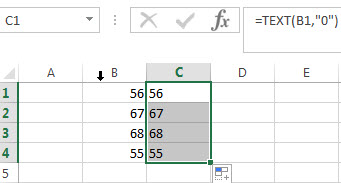You will see that all number are moved to the left side of their cells.

## Convert Numbers to Text with Format Cells Command

You can also use the Format Cells command to convert numbers to text in excel. Just refer to the following steps:

Step 1# Select the cells that you want to convert.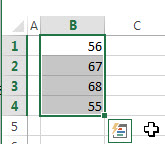Step2# Right click on the selected cells, and click “Format Cells…” from the drop-down list. Then the Format Cells dialog box will appear.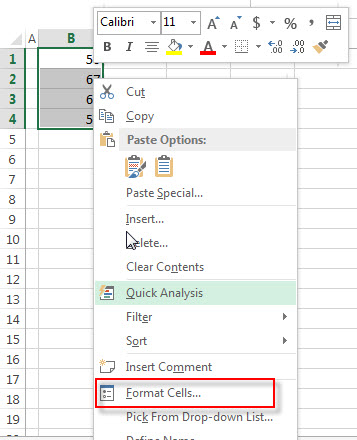Step3# switch to the Number Tab, then choose Text from the list of formatting categories.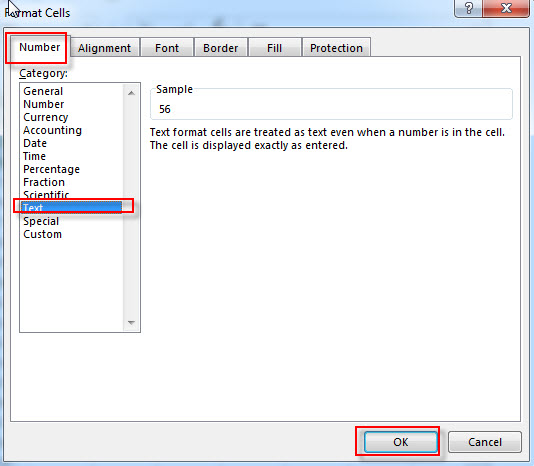Step4# click OK button.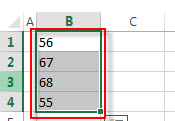You will see that the number in selected cells are converted as Text format.

## Convert Numbers to Text with concatenate operator

You can use the concatenate operator to join an empty text string and a number, then it will return a text string just containing the number. So it will be treated as Text string. So you can use the following formula to convert number in Cell B1 to text:

`=""&B1`

Now you can enter the above formula in Cell C1, just see the below screenshot: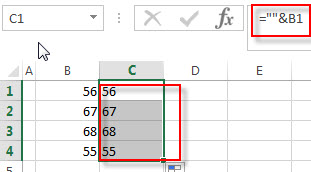### Related Functions

• Excel Concat function
The excel CONCAT function combines 2 or more strings or ranges together.This is a new function in Excel 2016 and it replaces the CONCATENATE function.The syntax of the CONCAT function is as below:=CONCAT (text1,[text2],…)…
• Excel Text function
The Excel TEXT function converts a numeric value into text string with a specified format. The TEXT function is a build-in function in Microsoft Excel and it is categorized as a Text Function. The syntax of the TEXT function is as below: = TEXT (value, Format code)…
Related Posts
How to Auto Fill Weekdays or Weekends in Excel

Sometimes we may want to enter a sequence of days in excel for some purpose, and we can press Ctrl+; to insert current date into cell, and then drag the cell down to attach following days into other cells, then ...

How to Change Date to The Day of Week in Excel

In excel, we can type a date properly if set cell format as ‘Date’. If there is a list includes multiple dates, is there any way to convert these dates to the days of week accordingly? Actually, there are some ...

How to Create Increment Number with Texts in Excel

Sometimes we want to fill 1,2,3,4,5… into each cell in a column, for implement this we can enter 1 in the first cell and then drag fill handle down to fill the following cells. But if there are some texts ...

How to Generate Random Time in Excel

In some special situations we may need to generate some random times in worksheet. We can enter time manually and one by one, but if we want to require a lot of random dates in excel worksheet, we will spend ...

How to Combine Text and Date into one Cell in Excel

This post will guide you how to combine text and dates in the same cell in Excel. How do I combine text and date values into one Cell in Excel 2013/2016. Combine Text and Date into One Cell Assuming that ...

How to Highlight Cell or Row If Date Is In Current Day/Week/Month in Excel

This post will guide you how to highlight cell if date is the current day or is in the current week or month in Excel. How do I highlight row if date is in current week or month with conditional ...

How to Convert Military Time to Standard Time in Excel

This post will guide you how to convert military time to standard time with a formula in Excel. How do I convert from military time 17:29:30 to regular time 5:29:30PM in Excel.  How do I Convert Standard time to military ...

How to Delete or Remove Year from a Date in Excel

This post will guide you how to delete or remove year from a given standard date in Excel. How do I remove the year from a date with a formula in Excel. Remove Year from a Date Assuming that you ...

How to Convert Date to YYYY-MM-DD format in Excel

This post will guide you how to convert the current date to a specified date format in Excel. How do I convert date to YYYY-MM-DD format with Format Cells Feature in Excel. How to convert date format to a specific ...

How to Concatenate Cells and keeping Date Format in Excel

This post will guide you how to concatenate cells and keeping data format in Excel. How do I concatenate cells and retain the certain Date format with a formula in Excel 2010/2013/2016. Concatenate Cells and Keeping Date Format Assuming that ...

Sidebar